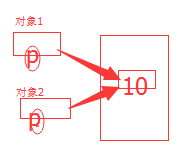# C++中构造函数、析构函数的执行顺序

1. 构造函数、析构函数概要
• 构造函数
调用顺序：
(1)全局对象的构造函数，
(2)main函数对象变量 ，包括static等依次执行
(3)main函数中的局部函数的局部对象成员变量
注意： static变量的构造函数只执行一次；
复合情况：按照声明顺序构造成员对象，由内向外
http://blog.csdn.net/xw13106209/article/details/6899370#t1
• 析构函数
执行顺序：
(1)退出函数时候，autostatic对象的析构函数，static变量仅执行一次；
(2)main函数，按照构造的逆序，析构automatic对象
(3)调用函数里面static对象析构函数
(4)调用main函数里面的static对象析构函数
(5)最后调用全局对象的析构函数
注意：复合情况：由外向内析构
2. 拷贝构造函数
三种情况下会执行拷贝函数。
(1) 使用某个对象对另外一个新对象进行初始化
(2)形参含有对象，在实参传值时候
(3)函数返回对象时候

两种情形下的析构函数：
(1)一般情况下，使用默认拷贝构造函数，仅仅是对新的对象成员进行赋值；

 A::A(const &r){
color = r.color; //所有成员都是直接赋值，而不执行其他的操作
num = r.num;
}

(2)手动编写拷贝构造函数：

class B{
static count = 0;
private int width;
B(int y){
width = y;
cout << "构造函数" ;
}
~B(){
cout --;
cout <<"析构函数";
}
}
// *******主函数*******
void main(){
B b(20);
B c(b);
}
//执行完后，我们会发现static为-1，理论上我们是想让static变成0，为神马变成-1呢？~~
//这时候我们发现程序运行时候，执行过程如下：
1 一次构造函数， 此时，count为1；
2 再执行一次拷贝函数,此时 count还是1；
3 最后程序结束时候 执行两次析构函数，而析构函数为同样的，都是count --，所以count变成-1；

B::B(B& r){
width = r.width;
cout ++;
cout << "拷贝构造函数";
}

class C{
private int *p ;

C(){
p = new int(5);
}
~C(){
delete p;
}
}
void main(){
C c ;
C c1(c);
}C::C(C& r){
p = new int(10);  //新分配空间
*p = *(r.p);   //赋值
}
1. 各个函数执行顺序
例子：
class A{
private int color;
private int num;
A(int x,int y){
color = x;
num = y;
cout << "构造A" <<endl;
}
A:A(int x,int y){
cout << "拷贝构造A" << endl;
}
~A(){
cout << "析构A" << endl;
}

}
A gloabl(0,0);  //全局变量
void main(){
static A a(1,1);
A b(a);//或者A b = a; (1)对象进行初始化

A prin(){//(2)返回对象
cout << "I Love China" <<endl;
}

void fub(A c){  //(3)实参传值
A n(11,2);
cout << "对象参数"<<endl;
}

prin();
fub(a);
cout("end");
}


// 构造A       全局变量globle
//构造A        static变量
//拷贝构造A    主函数 对象成员
//I Love China               prin函数
//拷贝构造A                   prin函数
//析构                        prin函数结束
//构造A               fub函数 对象成员
//对象参数            fub函数 输出内容
//拷贝构造A
//析构A
//析构A               fub函数结束
// end           主函数输出内容
//析构A   main函数的成员变量
//析构A   main函数的static变量
//析构A   全局变量

©️2019 CSDN 皮肤主题: 大白 设计师: CSDN官方博客